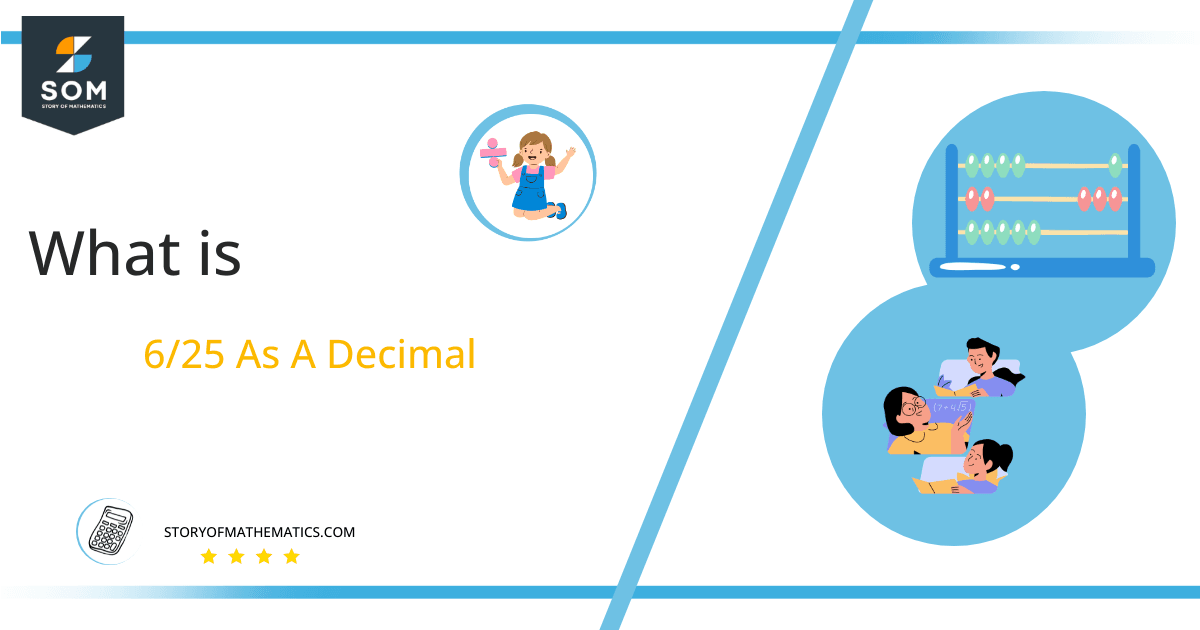# What Is 6/25 as a Decimal + Solution With Free StepsThe fraction 6/25 as a decimal is equal to 0.24.

In math, finance, and science, decimal numbers are often used to represent whole numbers and fractional parts. They come with an additional point that distinguishes them from regular powers of 10, which is the decimal place value system.

It can be challenging when we want to represent any quantity in terms of a fraction like 6/25 because the number will always sound awkward and not very repeatable. Therefore, a feasible solution is to convert it into its decimal equivalent. When represented as a decimal, the fraction 6/25 becomes more readily understandable.

Fractional conversions can be tricky, but converting a fraction into a decimal format is straightforward. This article will show you how and give plenty of examples so that transformation will not seem difficult.

Let us move forward to understand the long division method used to convert 6/25 into a decimal number.

## Solution

The method of expressing a fraction in decimal form is dividing its upper portion, the numerator, by the lower portion, called the denominator. The answer obtained as a decimal number is also called a quotient.

Further, this division in terms of dividend and divisor can be explained as:

Dividend = 6

Divisor = 25

The division for the fraction 6/25 will take place as follow:

Dividend ÷ Divisor = Quotient

6 ÷ 25 = 0.24

The detailed division is shown in the following figure 1:Figure 1

## 6/25 Long Division Method

The decimal equivalent of the given fraction can easily be found using modern calculators in seconds. Still, here we will learn the traditional long division method that saves us from errors and improves the mathematical calculations.

The division process is started by placing a decimal point at the quotient and adding a 0 to dividend to make it divisible by 25. The division results in:

60 ÷ 25 ≈ 2

Here, the remainder obtained is 10 as:

25 x 2 = 50

This shows that 60 – 50 gives ten; that is the remainder. Now proceeding with the division and adding another zero to 10 makes it 100. Therefore, the dividend then is 100 whereas the divisor is 25. Dividing 100 by 25 gives us:

100 ÷ 25 = 4

Since the remainder is zero, the decimal equivalent of the given fraction 6/25 is 0.24. The long division process helps to convert the given fraction easily into a decimal number. It also helps classify a given number as a terminating or nonterminating decimal.

The decimal equivalent of the given fraction is 0.24, a finite number, and none of the digits is repeating or recurring; therefore, the obtained decimal number is categorized as a terminating and non-repeating decimal number.Images/mathematical drawings are created with GeoGebra.